# The RHS Criterion - Proof

Go back to  'Triangles-Quadrilaterals'

Now, let us discuss a rigorous proof of the RHS criterion. Suppose that ∆ABC and ∆DEF are two right-angled triangles (right-angled at B and E respectively) such that AC = DF and AB = DE, as shown below: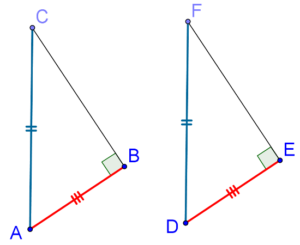If we were to show equality between ∠A and ∠D, or ∠C and ∠F, then we would be done (by the SAS criterion). Therefore, let us suppose that ∠A ≠ ∠D, and let us suppose that ∠A is greater: ∠A > ∠D.

Through A, draw AG (where G lies on BC) such that ∠BAG = ∠D, as show below: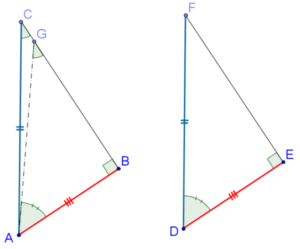By the ASA criterion, ∠ABG ≡ ∆DEF, which means that AG = DF. But DF is equal to AC (given to us), and so AG = AC.

Consider ∆AGC for a moment. We have shown that AG = AC, and thus the angles opposite these equal sides must be equal:

∠AGC = ∠ACG

However, we notice that ∠AGC is obtuse (it is the exterior angle for the right-angled ∆ABG). This would mean that we have two obtuse angles (∠AGC and ∠ACG) in the same triangle (∆AGC). Is this possible? Of course not!

Thus, our original assumption that ∠A ≠ ∠C is incorrect. The two angles are equal, and hence ∆ABC ≡ ∆DEF by the SAS criterion.

Example 1: If two triangles are congruent, prove that their respective

(a)  medians are equal

(b)  angle bisectors are equal

(c)  altitudes are equal

Solution: Although these results might seem obvious, let us still go ahead and prove them rigorously.

(a) In the following figure,  $$\Delta ABC \cong \Delta DEF$$ and AX and DY are a pair of (respective) medians, as shown: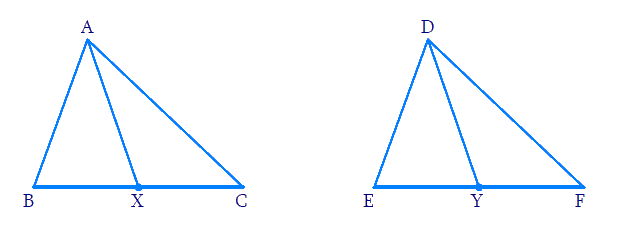Compare $$\Delta ABX\;and\;\Delta DEY.$$ We have:

• AB = DE

• $$BX = EY = \frac{1}{2}BC\;or\;\frac{1}{2}EF$$

• $$\angle B = \angle E$$

Clearly $$\Delta ABX \cong \Delta DEY$$ by the SAS criterion, so that AX = DY. Similarly, the other pairs of medians (respective) will be equal.

(b) In the following figure, $$\Delta ABC \cong \Delta DEF$$ and AX and DY are the angle bisectors of $$\angle A\;and\;\angle D$$ respectively: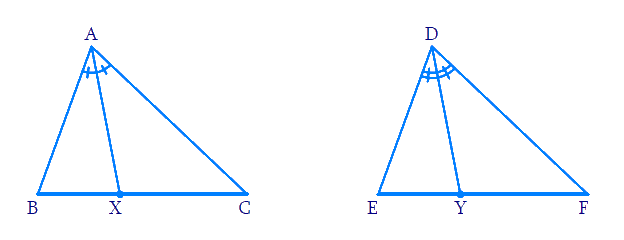Since $$\angle A\;and\;\angle D$$, we have $$\angle BAX$$=$$\angle EDY.$$ Also, $$\angle B\; = \;\angle E$$ which means that $$\angle ABX\; \cong \;\Delta DEY$$ by the ASA criterion.

Thus, AX = DY. Similarly, the other pairs of respective angle bisectors will be equal.

(c) In the following figure, AX and DY are the altitudes in (congruent) $$\Delta ABC$$ and $$\Delta DEF$$respectively: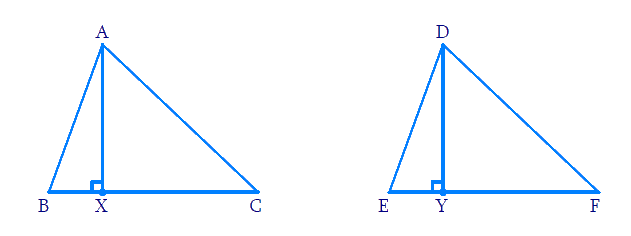Since $$AB = DE,\angle B = \angle E$$ and $$\angle BXA = \angle EYD,$$ we have $$\angle ABX \cong \Delta DEY$$ by the ASA criterion. Thus, AX = DY. And similarly, the other pairs of altitudes are equal.

These three results point to the fact that every parameter is (respectively) equal for two congruent triangles.

Example 2:    The bisector of $$\angle A$$ of $$\Delta ABC$$ is perpendicular to BC. What can you say about $$\angle ABC?$$

Solution:.  The answer to this problem might be evident to you directly. Consider the following figure: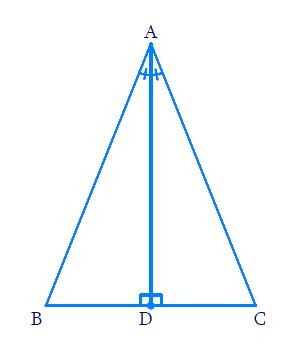Clearly, $$\angle ABD \cong \Delta ACD$$ by the ASA criterion, so that AB = AC. Thus, $$\angle ABC$$ is isosceles. Also, AD will be a median as well.